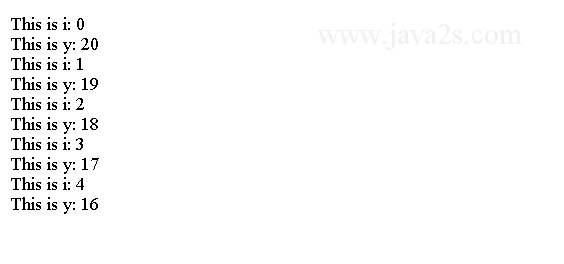# Java Tutorial - Java Hello World

Let's start by compiling and running the following short sample program.

```/* //from  w w w  .  j  ava  2s. c o m
This is a simple Java program. Call this file "Main.java".
*/

public class Main {
// Your program begins with a call to main().
public static void main(String args[]) {
System.out.println("Java.");
}
}
```

In Java, a source file is called a compilation unit. It is a text file that contains one or more class definitions. The Java compiler requires that a source file use the .java filename extension.

In Java, all code must reside inside a class. By convention, the name of the public class should match the its file name. And Java is case-sensitive.

The code above generates the following result.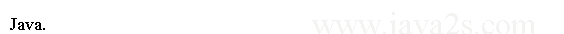## Compiling the Program

To compile the program, execute the compiler, `javac`, specifying the name of the source file on the command line:

```C:\>javac Main.java
```

The `javac` compiler creates a file called `Main.class`. `Main.class` contains the byte code version of the program.

To run the program, use the Java interpreter, called `java`. Pass the class name `Main` as a command-line argument, as shown here:

```C:\>java Main
```

When the program is run, the following output is displayed: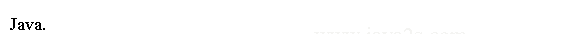When Java source code is compiled, each individual class is put into its own file named `classname.class`.

## A Closer Look at the Main.java

The first part is a comment.

```/*
This is a simple Java program. Call this file "Main.java".
*/
```

Comment is a remark for a program. The contents of a comment are ignored by the compiler. The next line of code in the program is shown here:

```public class Main {
```

The keyword `class` declares that a new class is being defined. `Main` is the name of the class. The entire class definition is between the opening curly brace (`{`) and the closing curly brace (`}`). The next line in the program is the single-line comment, shown here:

```// Your program begins with a call to main().
```

A single-line comment begins with a `//` and ends at the end of the line. The next line of code is shown here:

```public static void main(String args[]) {
```

Java applications begin execution by calling `main(String args[])`. Java is case-sensitive. Thus, `Main` is different from `main`.

## A Short Program with a variable

A variable is a memory location that may be assigned a value. The value of a variable is changeable.

The following code defines a variable and change its value by assigning a new value to it.

```public class Main {
public static void main(String args[]) {
int num; // a variable called num
num = 100;//  w  w w  .ja v a 2s  .com

System.out.println("This is num: " + num);
num = num * 2;

System.out.print("The value of num * 2 is ");
System.out.println(num);
}
}
```

When you run this program, you will see the following output: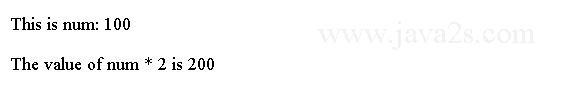The following snippet declares an integer variable called `num`. Java requires that variables must be declared before they can be used.

```int num; // this declares a variable called num
```

Following is the general form of a variable declaration:

```type var-name;
```

In the program, the line assigns to `num` the value 100.

```num = 100; // this assigns num the value 100
```

## Define more than one variable with comma

To declare more than one variable of the specified type, you may use a comma-separated list of variable names.

```public class Main {
public static void main(String args[]) {
int num, num2;
num = 100; // assigns num the value 100
num2 = 200;//from  w  w w.  j av a2 s  .c o  m
System.out.println("This is num: " + num);
System.out.println("This is num2: " + num2);

}
}
```

When the program is run, the following output is displayed:## Using Blocks of Code

Java can group two or more statements into blocks of code. Code block is enclosing the statements between opening and closing curly braces({}).

For example, a block can be a target for Java's `if` and `for` statements. Consider this `if` statement:

```public class Main {
public static void main(String args[]) {
int x, y;
x = 10;/* w  w w . ja  va  2 s  .c o m*/
y = 20;
if (x < y) { // begin a block
x = y;
y = 0;
System.out.println("x=" + x);
System.out.println("y=" + y);
} // end of block
}
}
```

Here is the output of the code above: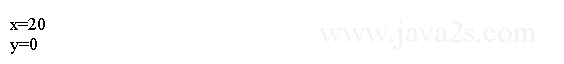## Example

A block of code as the target of a `for` loop.

```public class Main {
public static void main(String args[]) {
int i, y;//from  ww  w  .  j a  v  a  2  s. c o m
y = 20;
for (i = 0; i < 10; i++) { // the target of this loop is a block
System.out.println("This is i: " + i);
System.out.println("This is y: " + y);
y = y - 1;

}
}
}
```

The output generated by this program is shown here: